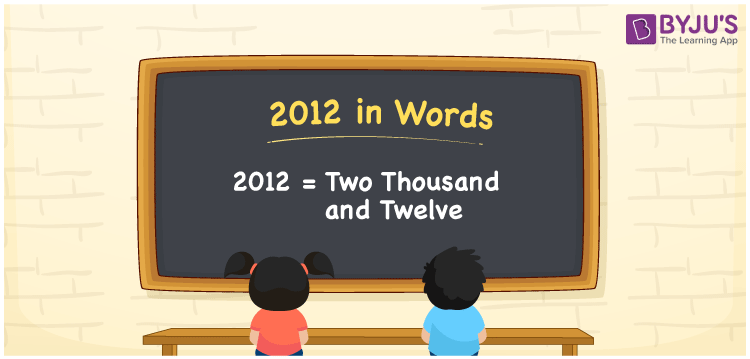# 2012 in Words

2012 in words is written as Two thousand twelve. In both the International System of Numerals and the Indian System of Numerals, 2012 is written as Two thousand twelve. The number 2012 is a Cardinal Number as it represents some quantity.

 2012 in Words Two thousand twelve Two thousand twelve in Number 2012

## 2012 in English Words

2012 in English words is read as “Two thousand twelve”.## How to Write 2012 in Words?

To write 2012 in words, we shall use the place value chart. In the place value chart, put 2 in the thousands, 0 in the hundreds, 1 in the tens, and 2 in the ones, respectively. Let us make a place value chart to write the number 2012 in words.

 Thousands Hundreds Tens Ones 2 0 1 2

Thus, we can write the expanded form as

2 × Thousand + 0 × Hundred + 1 × Ten + 2 × One

= 2 × 1000 + 0 × 100 + 1 × 10 + 2 × 1

= 2000 + 0 + 10 + 2

= 2012

= Two thousand twelve.

2012 is a natural number which is the successor of 2011 and the predecessor of 2013.

2012 in words – Two thousand twelve

• Is 2012 an odd number? – No
• Is 2012 an even number? – Yes
• Is 2012 a perfect square number? – No
• Is 2012 a perfect cube number? – No
• Is 2012 a prime number? – No
• Is 2012 a composite number? – Yes

## Frequently Asked Questions on 2012 in Words

Q1

### How to write 2012 in words?

2012 in words is written as Two thousand twelve.
Q2

### How to write 2012 in the International and Indian System of Numerals?

In both, the system of numerals, 2012 in words, is written as Two thousand twelve.
Q3

### How to write 2012 in a place value chart?

In the place value chart, write 2 in the thousands, 0 in the hundreds, 1 in the tens, and 2 in the ones, respectively.